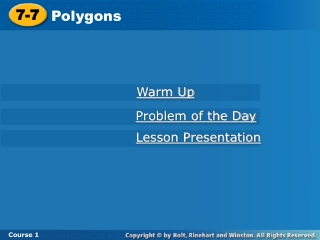DownloadDownload Presentation7-7

# 7-7

Télécharger la présentation## 7-7

- - - - - - - - - - - - - - - - - - - - - - - - - - - E N D - - - - - - - - - - - - - - - - - - - - - - - - - - -
##### Presentation Transcript

1. 7-7 Polygons Course 1 Warm Up Problem of the Day Lesson Presentation

2. 7-7 Polygons Course 1 Warm Up True or false? 1.Some trapezoids are parallelograms. 2. Some figures with 4 right angles are squares. false true

3. 7-7 Polygons Course 1 Problem of the Day Four square tables pushed together can seat either 8 or 10 people. How many people could 12 square tables pushed together seat? 14, 16, 18, or 26 people

4. 7-7 Polygons Course 1 Learn to identify regular and not regular polygons and to find the angle measures of regular polygons.

5. 7-7 Polygons Course 1 Insert Lesson Title Here Vocabulary polygon regular polygon

6. 7-7 Polygons Course 1 Triangles and quadrilaterals are examples of polygons. A polygon is a closed plane figure formed by three or more line segments. A regular polygon is a polygon in which all sides are congruent and all angles are congruent. Polygons are named by the number of their sides and angles.

7. 7-7 Polygons Course 1

8. 7-7 Polygons Course 1 Additional Example 1A: Identifying Polygons Tell whether each shape is a polygon. If so, give its name and tell whether it appears to be regular or not regular. A. The shape is a closed plane figure formed by 3 or more line segments. polygon There are 5 sides and 5 angles. pentagon All 5 sides do not appear to be congruent. Not regular

9. 7-7 Polygons Course 1 Additional Example 1B: Identifying Polygons Tell whether each shape is a polygon. If so, give its name and tell whether it appears to be regular or not regular. B. The shape is a closed plane figure formed by 3 or more line segments. polygon There are 8 sides and 8 angles. octagon The sides and angles appear to be congruent. regular

10. 7-7 Polygons Course 1 Try This: Example 1A Tell whether each shape is a polygon. If so, give its name and tell whether it appears to be regular or not regular. A. There are 4 sides and 4 angles. quadrilateral The sides and angles appear to be congruent. regular

11. 7-7 Polygons Course 1 Try This: Example 1B Tell whether each shape is a polygon. If so, give its name and tell whether it appears to be regular or not regular. B. There are 4 sides and 4 angles. quadrilateral All 4 sides do not appear to be congruent. regular

12. 7-7 Polygons Course 1 The sum of the interior angle measures in a triangle is 180°, so the sum of the interior angle measures in a quadrilateral is 360°.

13. 7-7 Polygons 1 Understand the Problem Course 1 Additional Example 2: Problem Solving Application Malcolm designed a wall hanging that was a regular 9-sided polygon (called a nonagon). What is the measure of each angle of the nonagon? • The answer will be the measure of each angle in a nonagon. • List the important information: • A regular nonagon has 9 congruent sides and 9 congruent angles.

14. 7-7 Polygons 3 Solve Make a Plan 2 Course 1 Additional Example 2 Continued Make a table to look for a pattern using regular polygons. Draw some regular polygons and divide each into triangles.

15. 7-7 Polygons 720° Course 1 Additional Example 2 Continued

16. 7-7 Polygons Course 1 Additional Example 2 Continued The number of triangles is always 2 fewer than the number of sides. A nonagon can be divided into 9 – 2 = 7 triangles. The sum of the interior angle measures in a nonagon is 7  180° = 1,260°. So the measure of each angle is 1,260° ÷ 9 = 140°.

17. 7-7 Polygons 4 Course 1 Additional Example 2 Continued Look Back Each angle in a nonagon is obtuse. 140° is a reasonable answer, because an obtuse angle is between 90° and 180°.

18. 7-7 Polygons 1 Understand the Problem Course 1 Try This: Additional Example 2 Sara designed a picture that was a regular 6-sided polygon (called a hexagon). What is the measure of each angle of the hexagon? • The answer will be the measure of each angle in a hexagon. • List the important information: • A regular hexagon has 6 congruent sides and 6 congruent angles.

19. 7-7 Polygons 3 Solve Make a Plan 2 Course 1 Try This: Example 2 Continued Make a table to look for a pattern using regular polygons. Draw some regular polygons and divide each into triangles.

20. 7-7 Polygons Course 1 Try This: Example 2 Continued

21. 7-7 Polygons Course 1 Try This: Example 2 Continued The number of triangles is always 2 fewer than the number of sides. A hexagon can be divided into 6 – 2 = 4 triangles. The sum of the interior angles in a octagon is 4  180° = 720°. So the measure of each angle is 720° ÷ 6 = 120°.

22. 7-7 Polygons 4 Course 1 Try This: Example 2 Continued Look Back Each angle in a hexagon is obtuse. 120° is a reasonable answer, because an obtuse angle is between 90° and 180°.

23. 7-7 Polygons Course 1 Insert Lesson Title Here Lesson Quiz 1. Name each polygon and tell whether it appears to be regular or not regular. 2. What is the measure of each angle in a regular dodecagon (12-sided figure)? nonagon, regular; octagon, not regular 150°